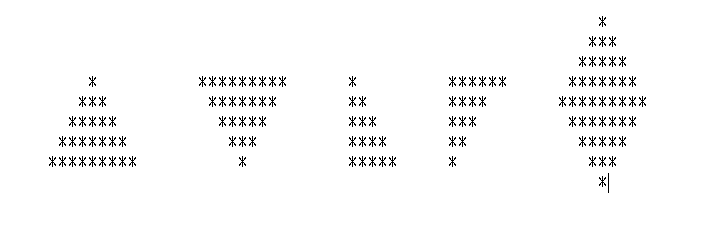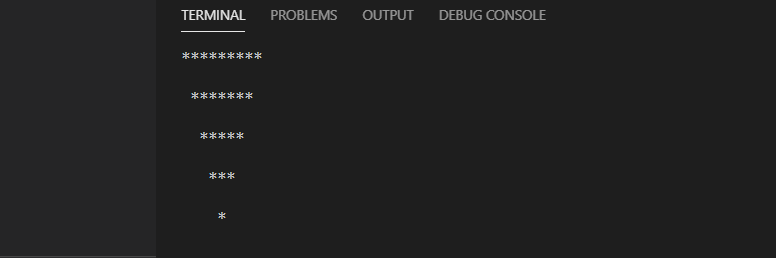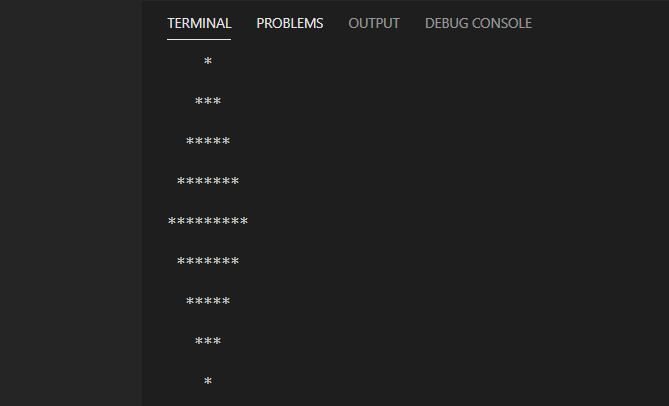# [Java basics] Let's make a triangle with a for statement

This is a problem that my senior at the company gave to me as a newcomer to make various triangles using for statements in Java. This is Java, but you can make it with a simple for statement, so please try other languages as well.

The shape to be handled is as shown in the image below.## Target audience

・ Those who are studying programming ・ Those who have recently become engineers ・ Those who want to study Java

# Problem ① Equilateral triangle ▲

``````public class Main {
public static void main(String[] args) {
int max = 5;
String star = "*";

for (int i = 0; i < max; i++) {
for(int j = 0; j < max-i; j++) {
System.out.print(" ");
}
for(int k = 0; k <= (i*2); k++) {
System.out.print(star);
}
System.out.println("\n");
}
}
}
``````

## Output result## Commentary

-Define the height of the triangle in the first for statement (i) (this time, the variable max = 5 is set in advance). -Define a blank ("") in the second for statement (j). Since it is a triangle, the blanks are the maximum at first, and the blanks are gradually reduced in inverse proportion to the number of *. The conditional expression is j <max-i ;. -Output * with the third for statement (k). -Finally, every time one loop ends, println will start a new line. (If you do not start a new line, it will be output horizontally all the time)

# Problem ② Inverted triangle ▼

``````public class Main {
public static void main(String[] args) {
int max = 5;
String star = "*";

for (int i = 0; i < max; i++) {
for(int j = 0; j < i; j++) {
System.out.print(" ");
}
for(int k = 0; k <= max*2-(i*2)-2; k++){
System.out.print(star);
}
System.out.println("\n");
}
}
}
``````

## Output result## Commentary

・ Define the height of the triangle in the first for statement (i) -Define a blank ("") in the second for statement (j). Unlike ①, this time I will simply increase it by 1. -Output * with the third for statement (k).

• I'm solving it by pushing ... Please tell me if you have a better hand ... orz

# Problem ③ Right triangle ver.1 ◣

``````public class Main {
public static void main(String[] args) {
int max = 5;
String star = "*";

for (int i = 0; i < max; i++) {
for (int j = 0; j <= i; j++) {
System.out.print(star);
}
System.out.println("\n");
}
}
}
``````

## Output result## Commentary

This is probably the simplest.

・ Define the height of the triangle in the first for statement (i) -Output * so that it increases by one in the second for statement (j).

# Problem ④ Right triangle ver.2 ◢

``````public class Main {
public static void main(String[] args) {
int max = 5;
String star = "*";

for (int i = 0; i < max; i++) {
for (int j = 0; j < max-i; j++) {
System.out.print(" ");
}
for (int k = 0; k <= i; k++){
System.out.print(star);
}
System.out.println("\n");
}
}
}
``````

## Output result## Commentary

I think this is easy if you can make an inverted triangle.

・ Define the height of the triangle in the first for statement (i) -Define a blank ("") in the second for statement (j). At first, the blank is the maximum, and the number of blanks is gradually reduced in inverse proportion to the number of *. The conditional expression is j <max-i ;. -Output * with the third for statement (k). Since it is incremented one by one, k <= i.

# Problem ⑤ Rhombus ◇

``````public class Main {
public static void main(String[] args) {
int max = 5;
String star = "*";

for (int i = 0; i < max; i++) {
for (int j = 0; j < max-i; j++) {
System.out.print(" ");
}
for (int k = 0; k <= ((i-1)*2); k++) {
System.out.print(star);
}
System.out.println("\n");
if (i == 4){
for (int a = 0; a < max; a++) {
for(int b = 0; b < a; b++) {
System.out.print(" ");
}
for(int c = 0; c <= max*2-(a*2)-2; c++){
System.out.print(star);
}
System.out.println("\n");
}
}
}
}
}
``````

## Output result## Commentary

I thought about solving this for a while. As a result, use the if statement to create both equilateral and inverted triangles. I used the hand of outputting, but I think there is probably a better way ...

First, output from 1 to 7 * by the method of an equilateral triangle, and express by an inverted triangle from 9 to 1 *. Let's set if (i == 4) and switch between ▲ and ▼ when the i loop turns 4 times.

The rest can be solved by reusing the codes ① to ④.

## Summary

I summarized how to output various shapes using the for statement. The for statement is frequent in practice, and it will be a practice to think about the structure of the code, so please try it (^^) /